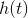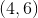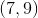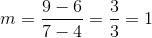# GMAT Math : DSQ: Graphing a function

## Example Questions

### Example Question #1 : Dsq: Graphing A Function

Find the graph of the linear function.

I)passes through the pointsand.

II)intercepts the-axis at.

Both statements are needed to answer the question.

Statement II is sufficient to answer the question, but statement I is not sufficient to answer the question.

Statement I is sufficient to answer the question, but statement II is not sufficient to answer the question.

Either statement is sufficient to answer the question.

Statement I is sufficient to answer the question, but statement II is not sufficient to answer the question.

Explanation:

Find the graph of the linear function.

I)passes through the pointsand.

II)intercepts the-axis at.

Using I), we can find the slope of the function, and then we can start at either point and extend the slope in either direction to find our graph:So, using I) we are able to find the slope, from which we can find our graph

II) gives us one point, but without any more information, we cannot use II) by itself to find the rest of the graph

So:

Statement I is sufficient to answer the question, but statement II is not sufficient to answer the question.